# Linear Equations in Two Variables Graphing Linear Equations

• Slides: 89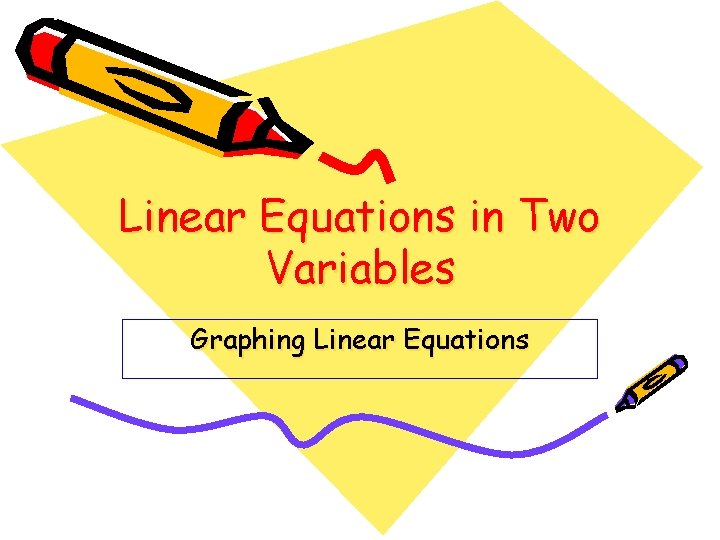Linear Equations in Two Variables Graphing Linear Equations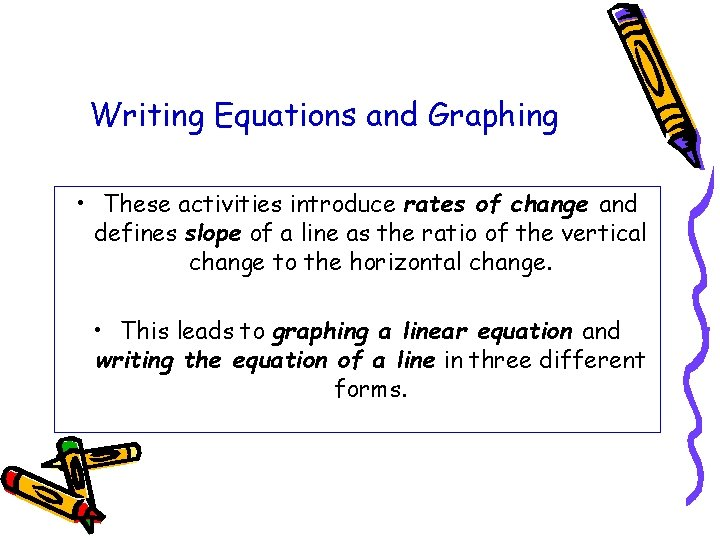Writing Equations and Graphing • These activities introduce rates of change and defines slope of a line as the ratio of the vertical change to the horizontal change. • This leads to graphing a linear equation and writing the equation of a line in three different forms.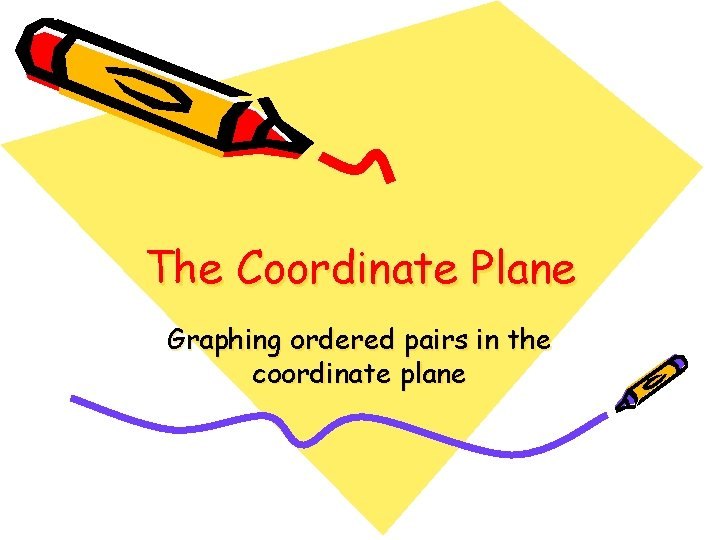The Coordinate Plane Graphing ordered pairs in the coordinate planeCoordinate axes origin quadrants ordered pair The coordinate plane is formed by placing two number lines called coordinate axes so that one is horizontal (x-axis) and one is vertical (y-axis). These axes intersect at a point called the origin (0, 0), which is labeled 0. An ordered pair (x, y) is a pair of real numbers that correspond to a point in the coordinate plane. The first number in an ordered pair is the x-coordinate and the second number is the y-coordinate.In a previous lesson, you learned to plot points from a table of values. The solution to an equation in two variables is all ordered pairs of real numbers (x, y) that satisfy the equation. You can graph the solution to an equation in two variables on the coordinate plane by using the (x, y) values from the table. Graph each ordered pair in the table. Do all the points appear to lie on the line?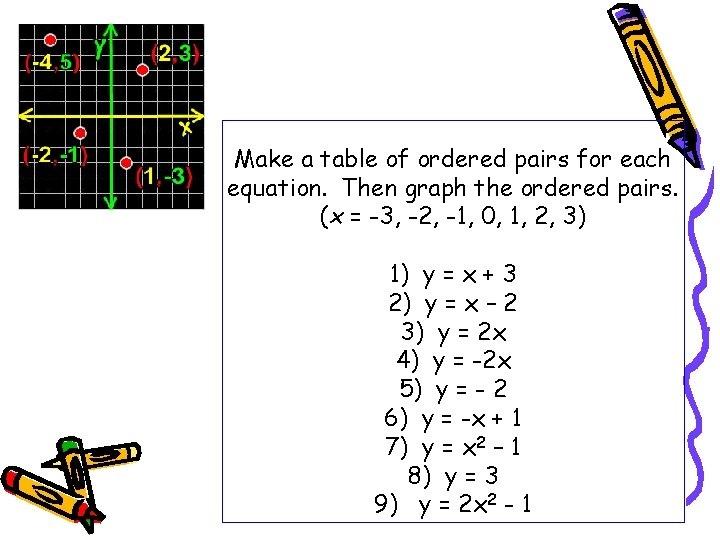Make a table of ordered pairs for each equation. Then graph the ordered pairs. (x = -3, -2, -1, 0, 1, 2, 3) 1) y = x + 3 2) y = x – 2 3) y = 2 x 4) y = -2 x 5) y = - 2 6) y = -x + 1 7) y = x 2 – 1 8) y = 3 9) y = 2 x 2 - 1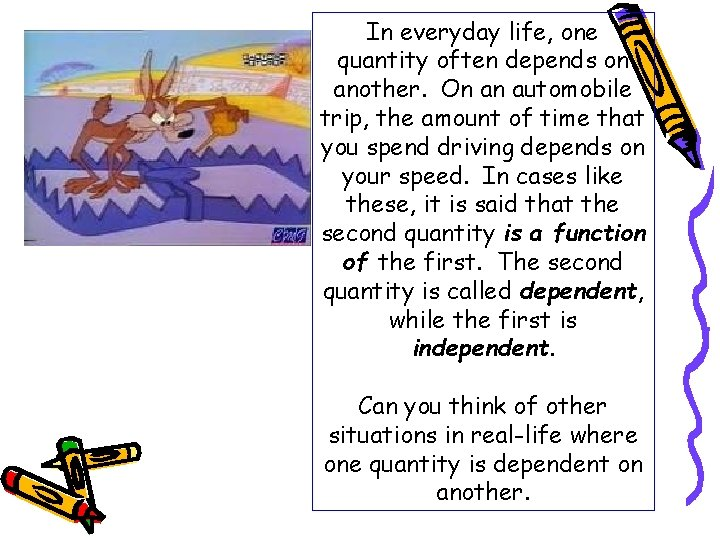In everyday life, one quantity often depends on another. On an automobile trip, the amount of time that you spend driving depends on your speed. In cases like these, it is said that the second quantity is a function of the first. The second quantity is called dependent, while the first is independent. Can you think of other situations in real-life where one quantity is dependent on another.Many functions (equation) can be represented by an equation in two variables. The variable that represents the dependent quantity is called the dependent variable. The variable that represents the independent quantity is called the independent variable. When you write an ordered pair using these variables (x, y), the independent variable appears first. (independent, dependent)In the function y = 2 x, each x-value is paired with exactly one y-value. Thus the value of y depends on the value of x. In a function, the variable of the domain is called the independent variable and the variable of the range is called the dependent variable. On a graph, the independent variable is represented on the horizontal axis and the dependent variable is represented on the vertical axis.Example The value v (in cents) of n nickels is given by the function (equation) v = 5 n a) Identify the independent and dependent variable. b) Represent this function in a table for n = 0, 1, 2, 3, 4, 5, 6. c) Represent this function in a graph.Domain Range Use both a table and a graph to represent each function for the given values of the independent variable. 1) The value v of 0, 1, 2, 3, 4, 5, and 6 dimes is a function of the number of dimes, d. 2) The distance d traveled by a jogger who jogs 0. 1 miles per minute over 0, 1, 2, 3, 4, and 5 minutes is a function of time, t. Try another 3) One hat cost \$24. The total cost c of 1, 2, 3, 4, 5, and 6 hats is a function of the number n of hats purchased.A 1200 -gallon tank is empty. A valve is opened and water flows into the tank at the constant rate of 25 gallons per minute. 1) How would you find the amount of water in the tank after 5 minutes, 6 minutes, and 7 minutes? 2) How would you find the time it takes for the tank to contain 500 gallons, 600 gallons, and 700 gallons? What is the independent variable? What is the dependent variable? What function represents this situation?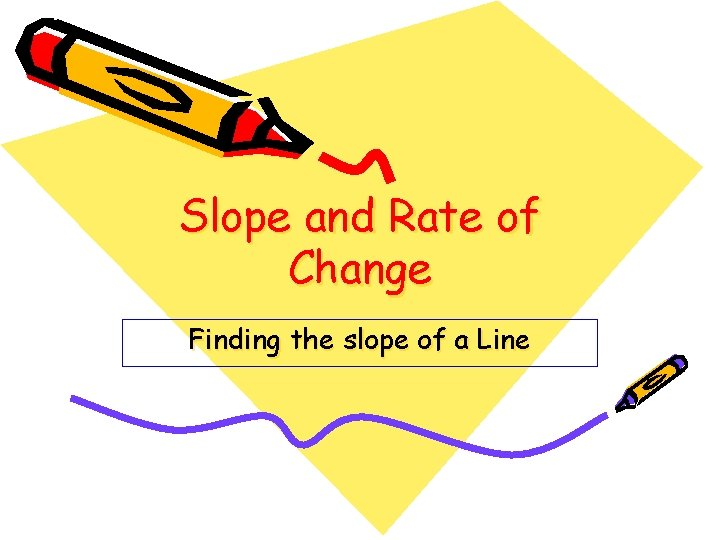Slope and Rate of Change Finding the slope of a LineSlope – A measure of the steepness of a line on a graph; rise divided by the run. If P (x 1, y 1) and Q (x 2, y 2) lie along a nonvertical line in the coordinate plane, the line has slope m, given by Linear equation – An equation whose solutions form a straight line on a coordinate plane. Collinear – Points that lie on the same line.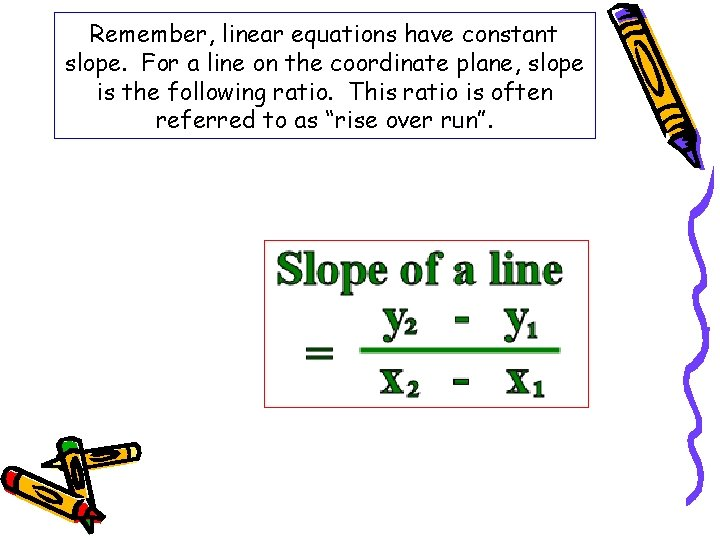Remember, linear equations have constant slope. For a line on the coordinate plane, slope is the following ratio. This ratio is often referred to as “rise over run”.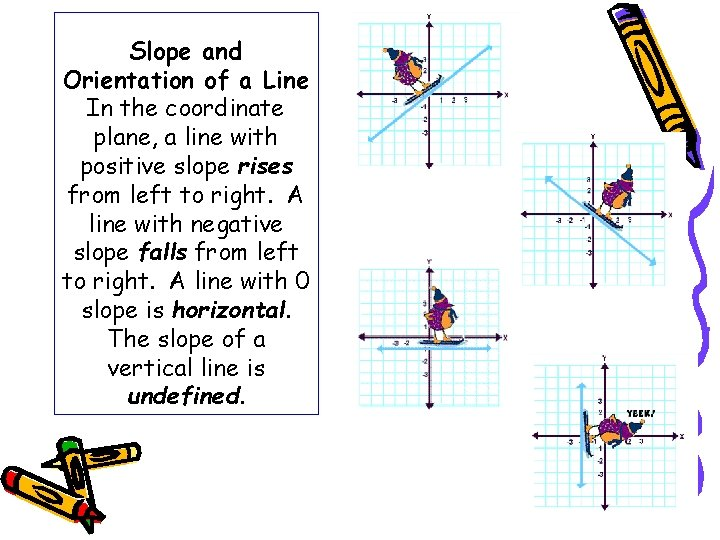Slope and Orientation of a Line In the coordinate plane, a line with positive slope rises from left to right. A line with negative slope falls from left to right. A line with 0 slope is horizontal. The slope of a vertical line is undefined.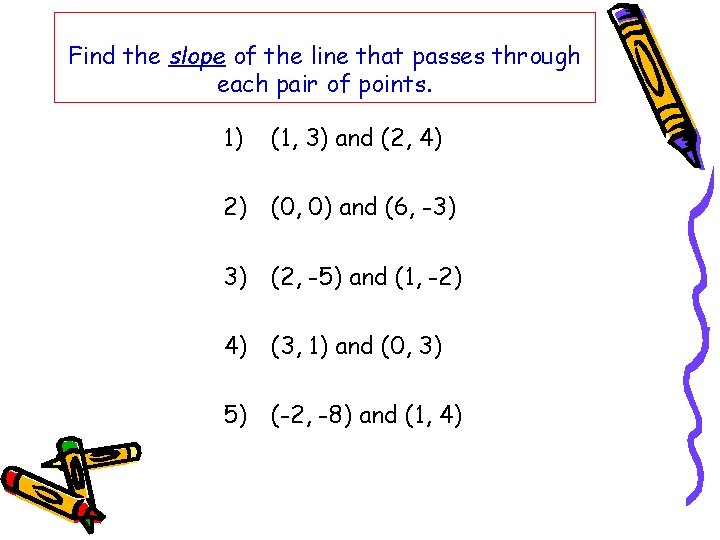Find the slope of the line that passes through each pair of points. 1) (1, 3) and (2, 4) 2) (0, 0) and (6, -3) 3) (2, -5) and (1, -2) 4) (3, 1) and (0, 3) 5) (-2, -8) and (1, 4)Finding points on a Line Graphing a line using a point and the slope is shown here. Graph the line passing through (1, 3) with slope 2. a) Graph point (1, 3). b) Because the slope is 2, you can find a second point on the line by counting up 2 units and then right 1 unit. C) Draw a line through point (1, 3) and the new point.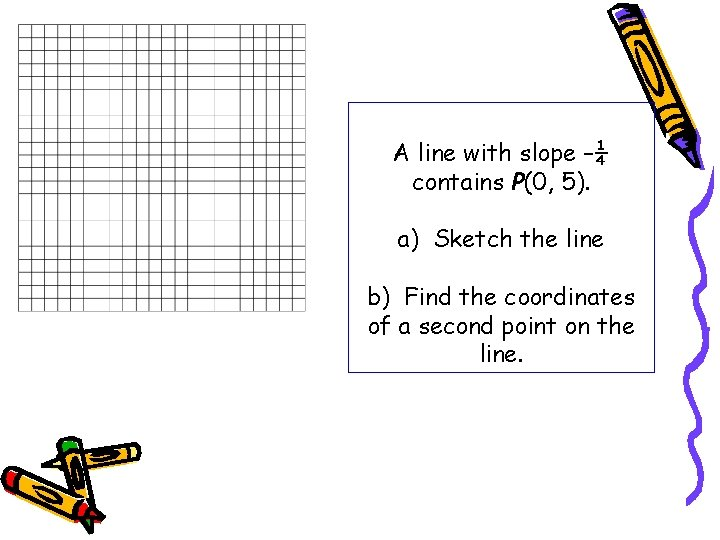A line with slope –¼ contains P(0, 5). a) Sketch the line b) Find the coordinates of a second point on the line.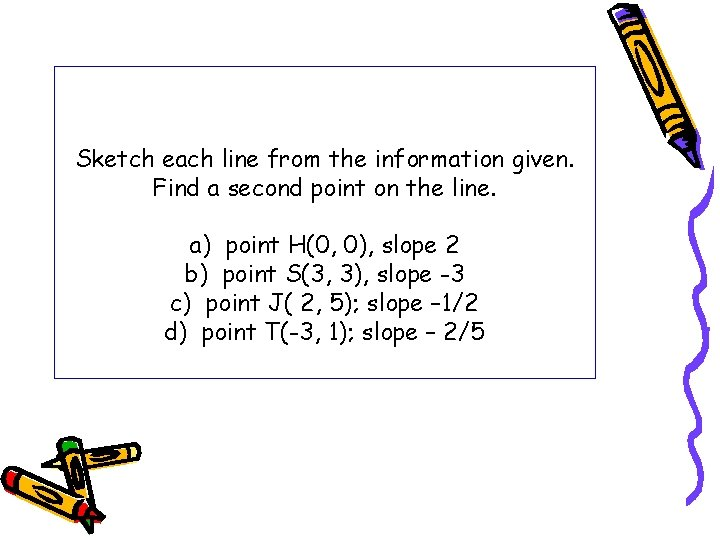Sketch each line from the information given. Find a second point on the line. a) point H(0, 0), slope 2 b) point S(3, 3), slope -3 c) point J( 2, 5); slope – 1/2 d) point T(-3, 1); slope – 2/5Finding Slope from a Graph Choose two points on the line (-4, 4) and (8, -2). Count the rise over run or you can use the slope formula. Notice if you switch (x 1, y 1) and (x 2, y 2), you get the same slope:Real-world Applications You have been solving problems in which quantities change since early school days. For instance, here is a typical first-grade problem: If I have two apples, and then I buy five more apples, how many apples do I have in all? This situation concerns a change in the number of apples. Try this problemOne marker on a road showed 20 miles. The next marker showed 170 miles. How many miles have gone by? The time was 2: 00 pm at the first marker. Then it was 5: 00 pm at the second marker. How may hours have passed? 150/3 compares quantities and is called rate. As you can see, simple subtraction is all that is required to answer the questions. The answers, 150 miles and 3 hours, indicate a change in miles and a change in time, respectively.Since each quantity describes a change, the expression is a rate of change. You read the rate as 150 miles in 3 hours or 150 miles per 3 hours. Rates can be positive (+) or negative (-) In everyday life, most rates are given “per one unit” of a quantity. This is called a unit rate. So, it is more common to perform the division 150/3 = 50 to arrive at a unit rate of change: 50 miles per 1 hour or 50 miles per hour.After stopping to buy gas, a motorist drives at a constant rate as indicated below. Time (t) 0 hrs Dist (d) 5 mi 2 hrs 120 mi Find the speed or rate of change for the car then graph the situation. Problem solving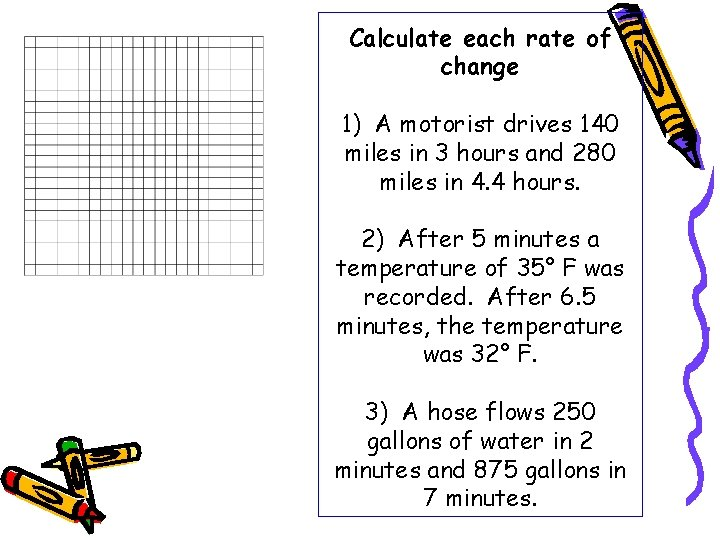Calculate each rate of change 1) A motorist drives 140 miles in 3 hours and 280 miles in 4. 4 hours. 2) After 5 minutes a temperature of 35° F was recorded. After 6. 5 minutes, the temperature was 32° F. 3) A hose flows 250 gallons of water in 2 minutes and 875 gallons in 7 minutes.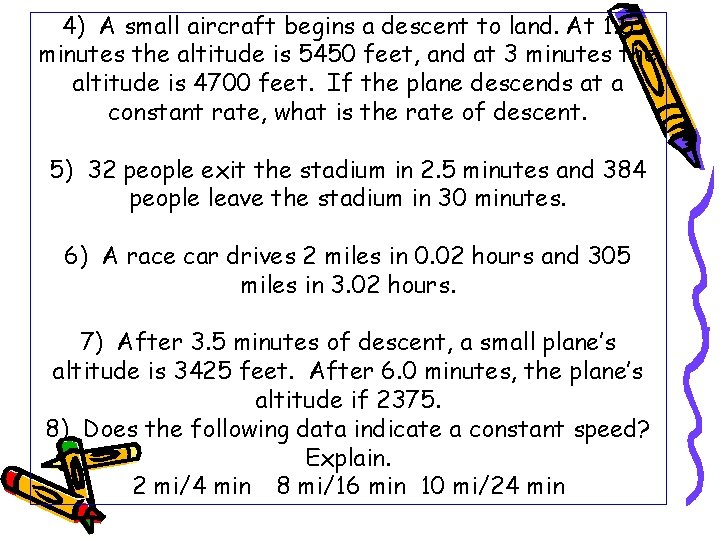4) A small aircraft begins a descent to land. At 1. 5 minutes the altitude is 5450 feet, and at 3 minutes the altitude is 4700 feet. If the plane descends at a constant rate, what is the rate of descent. 5) 32 people exit the stadium in 2. 5 minutes and 384 people leave the stadium in 30 minutes. 6) A race car drives 2 miles in 0. 02 hours and 305 miles in 3. 02 hours. 7) After 3. 5 minutes of descent, a small plane’s altitude is 3425 feet. After 6. 0 minutes, the plane’s altitude if 2375. 8) Does the following data indicate a constant speed? Explain. 2 mi/4 min 8 mi/16 min 10 mi/24 minA linear equation is an equation whose solutions fall on a line on the coordinate plane. All solutions of a particular linear equation fall on the line, and all the points on the line are solutions of the equation. Look at the graph to the left, points (1, 3) and (-3, -5) are found on the line and are solutions to the equation.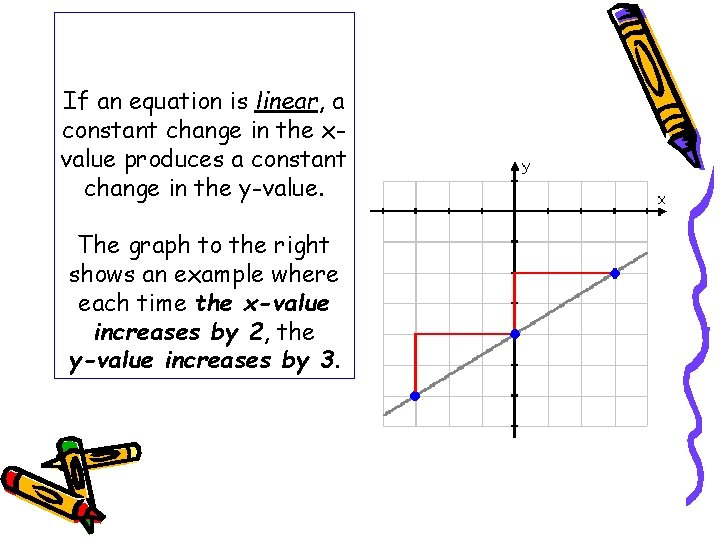If an equation is linear, a constant change in the xvalue produces a constant change in the y-value. The graph to the right shows an example where each time the x-value increases by 2, the y-value increases by 3.The equation y = 2 x + 6 is a linear equation because it is the graph of a straight line and each time x increases by 1 unit, y increases by 2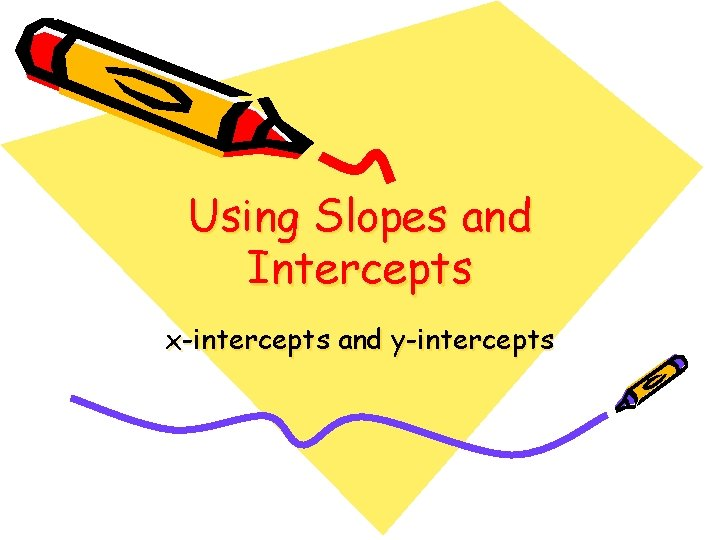Using Slopes and Intercepts x-intercepts and y-intercepts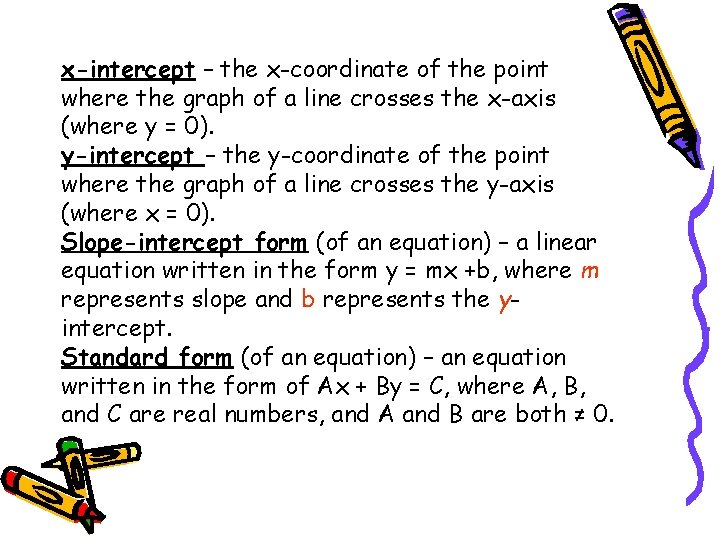x-intercept – the x-coordinate of the point where the graph of a line crosses the x-axis (where y = 0). y-intercept – the y-coordinate of the point where the graph of a line crosses the y-axis (where x = 0). Slope-intercept form (of an equation) – a linear equation written in the form y = mx +b, where m represents slope and b represents the yintercept. Standard form (of an equation) – an equation written in the form of Ax + By = C, where A, B, and C are real numbers, and A and B are both ≠ 0.Standard Form of an Equation • The standard form of a linear equation, you can use the x- and yintercepts to make a graph. • The x-intercept is the x-value of the point where the line crosses. • The y-intercept is the y-value of the point where the line crosses. Ax + By = CTo graph a linear equation in standard form, you fine the x-intercept by substituting 0 for y and solving for x. Then substitute 0 for x and solve for y. 2 x + 3 y = 6 2 x + 3(0) = 6 2 x = 6 x=3 2 x + 3 y = 6 2(0) + 3 y = 6 y=2 The x-intercept is 3. (y = 0) The y-intercept is 2. (x = 0)Find the x-intercept and y-intercept of each line. Use the intercepts to graph the equation. 1) x–y=5 2) 2 x + 3 y = 12 3) 4 x = 12 + 3 y 4) 2 x + y = 7 5) 2 y = 20 – 4 xSlope-intercept Form y = mx + b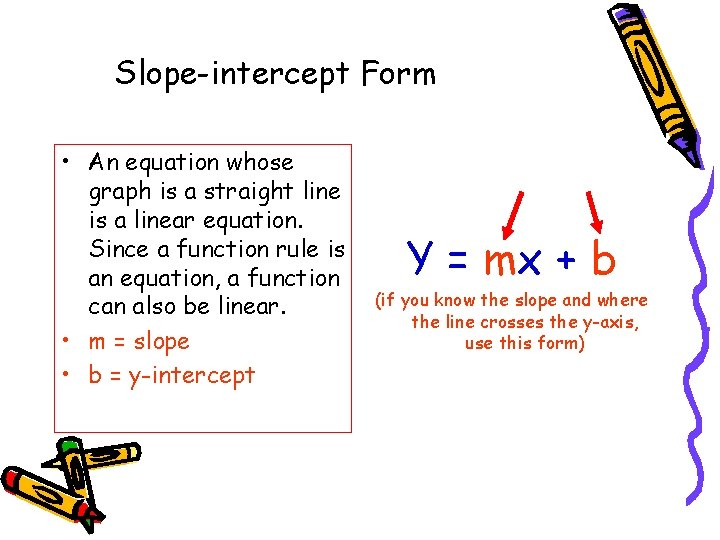Slope-intercept Form • An equation whose graph is a straight line is a linear equation. Since a function rule is an equation, a function can also be linear. • m = slope • b = y-intercept Y = mx + b (if you know the slope and where the line crosses the y-axis, use this form)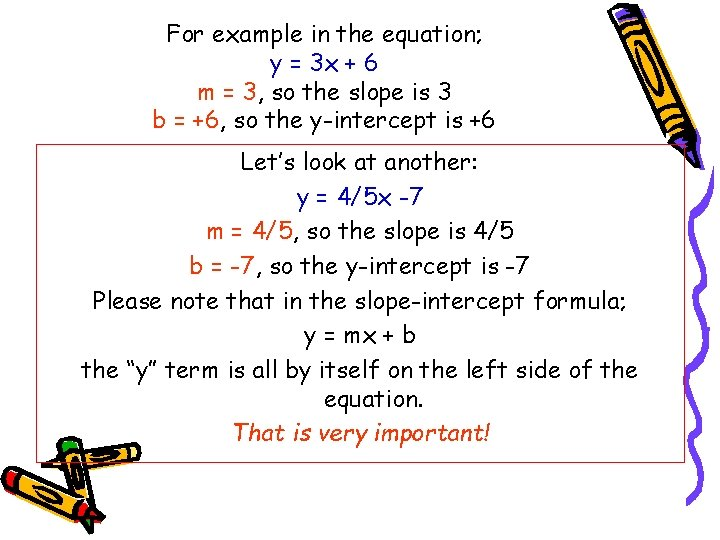For example in the equation; y = 3 x + 6 m = 3, so the slope is 3 b = +6, so the y-intercept is +6 Let’s look at another: y = 4/5 x -7 m = 4/5, so the slope is 4/5 b = -7, so the y-intercept is -7 Please note that in the slope-intercept formula; y = mx + b the “y” term is all by itself on the left side of the equation. That is very important!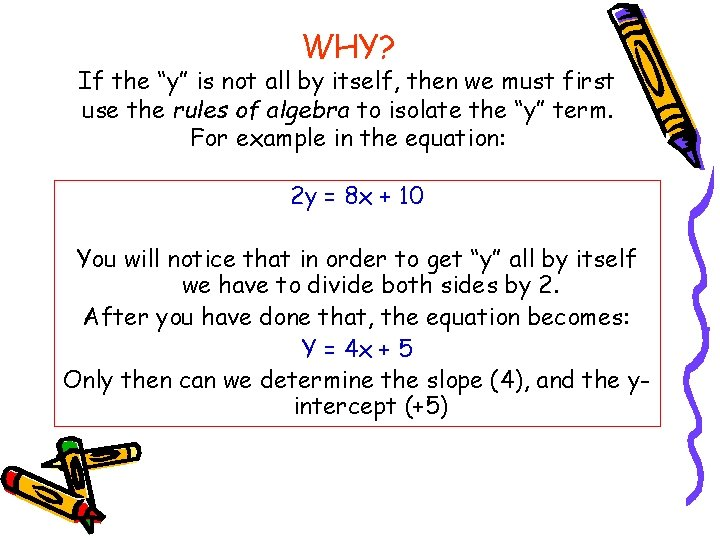WHY? If the “y” is not all by itself, then we must first use the rules of algebra to isolate the “y” term. For example in the equation: 2 y = 8 x + 10 You will notice that in order to get “y” all by itself we have to divide both sides by 2. After you have done that, the equation becomes: Y = 4 x + 5 Only then can we determine the slope (4), and the yintercept (+5)OK…getting back to the lesson… Your job is to write the equation of a line after you are given the slope and y-intercept… Let’s try one… Given “m” (the slope remember!) = 2 And “b” (the y-intercept) = +9 All you have to do is plug those values into y = mx + b The equation becomes… y = 2 x + 9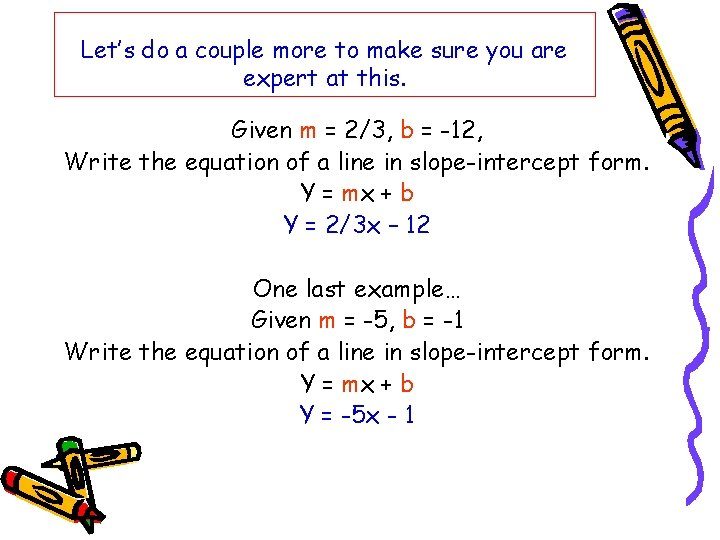Let’s do a couple more to make sure you are expert at this. Given m = 2/3, b = -12, Write the equation of a line in slope-intercept form. Y = mx + b Y = 2/3 x – 12 One last example… Given m = -5, b = -1 Write the equation of a line in slope-intercept form. Y = mx + b Y = -5 x - 1Writing an Equation From a Graph You can write an equation from a graph. Use 2 points to find the slope. Then use the slope and the yintercept to write the equation. Step 1 Using the slope formula, find the slope. Two points on the line are (0, 2) and (4, -1). Step 2 write an equation in slopeintercept form. The y-intercept is 2.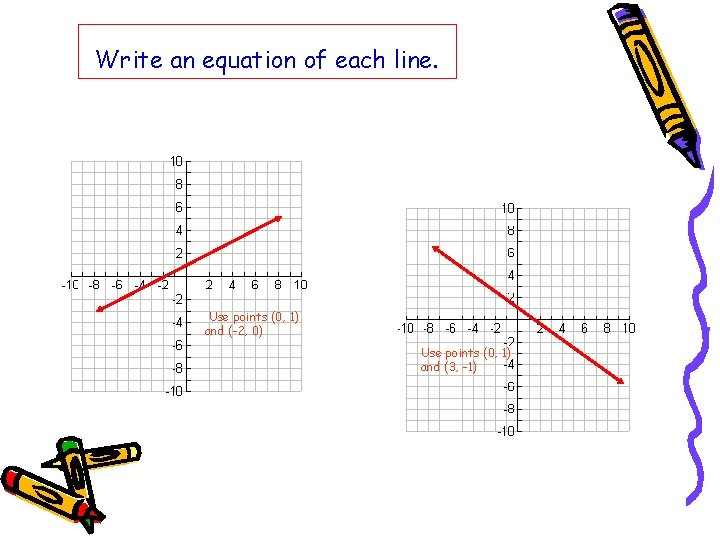Write an equation of each line. Use points (0, 1) and (-2, 0) Use points (0, 1) and (3, -1)Given the slope and y-intercept, write the equation of a line in slope-intercept form. 1) m = 3, b = -14 2) m = -½, b = 4 3) m = -3, b = -7 4) m = 1/2 , b = 0 5) m = 2, b = 4Using slope-intercept form to find slopes and y-intercepts The graph at the right shows the equation of a line both in standard form and slope-intercept form. You must rewrite the equation 6 x – 3 y = 12 in slope-intercept to be able to identify the slope and yintercept.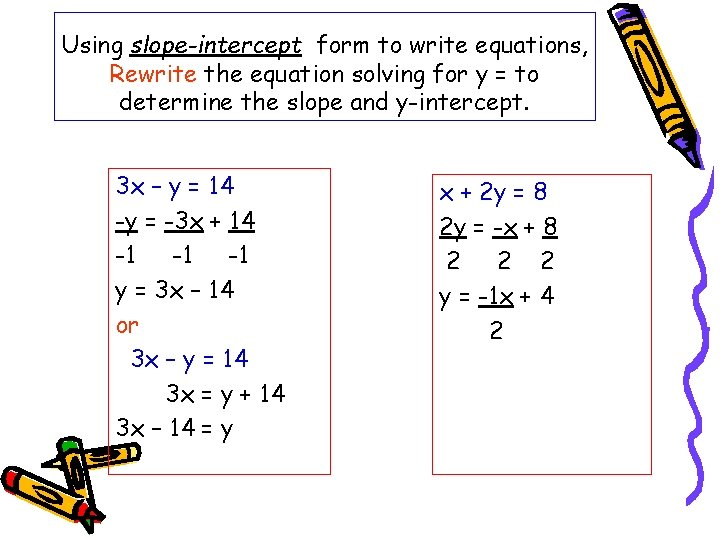Using slope-intercept form to write equations, Rewrite the equation solving for y = to determine the slope and y-intercept. 3 x – y = 14 -y = -3 x + 14 -1 -1 -1 y = 3 x – 14 or 3 x – y = 14 3 x = y + 14 3 x – 14 = y x + 2 y = 8 2 y = -x + 8 2 2 2 y = -1 x + 4 2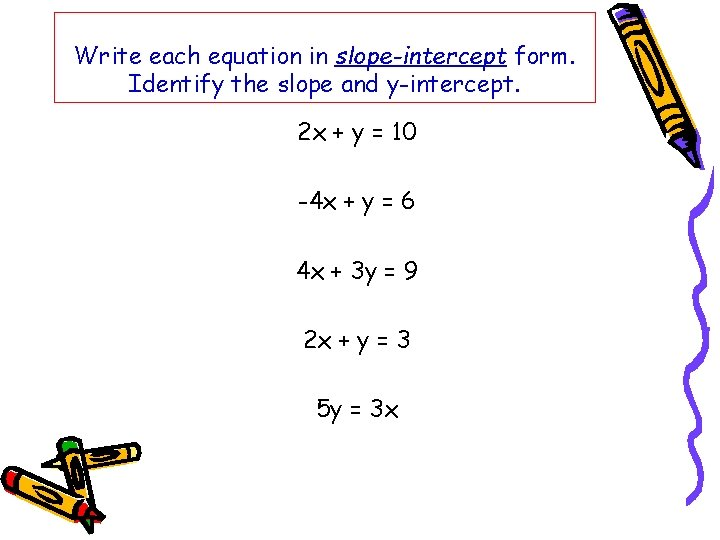Write each equation in slope-intercept form. Identify the slope and y-intercept. 2 x + y = 10 -4 x + y = 6 4 x + 3 y = 9 2 x + y = 3 5 y = 3 xWrite the equation of a line in slope-intercept form that passes through points (3, -4) and (-1, 4). 1) Find the slope. 4 – (-4) 8 -1 – 3 -4 m = -2 2) Choose either point and substitute. Solve for b. y = mx + b (3, -4) -4 = (-2)(3) + b -4 = -6 + b 2=b Substitute m and b in equation. Y = mx + b Y = -2 x + 2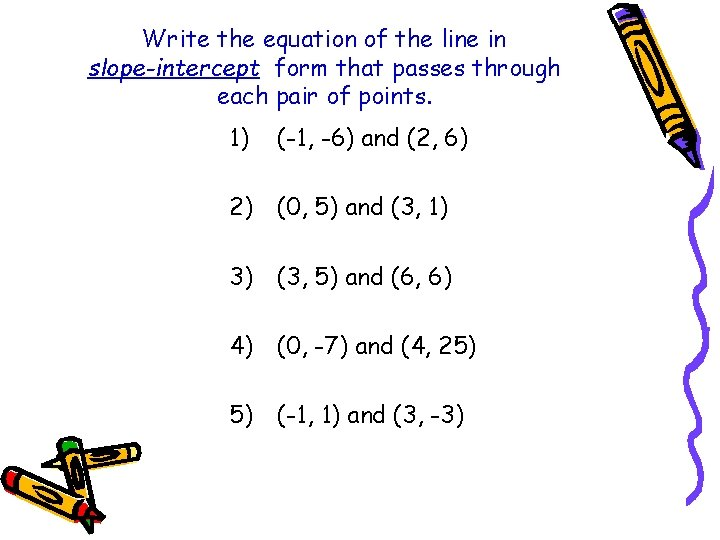Write the equation of the line in slope-intercept form that passes through each pair of points. 1) (-1, -6) and (2, 6) 2) (0, 5) and (3, 1) 3) (3, 5) and (6, 6) 4) (0, -7) and (4, 25) 5) (-1, 1) and (3, -3)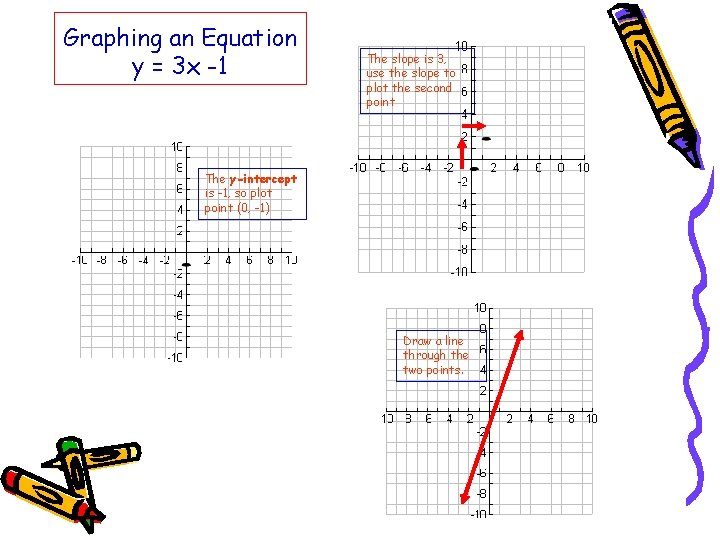Graphing an Equation y = 3 x -1 The slope is 3, use the slope to plot the second point The y-intercept is -1, so plot point (0, -1) Draw a line through the two points.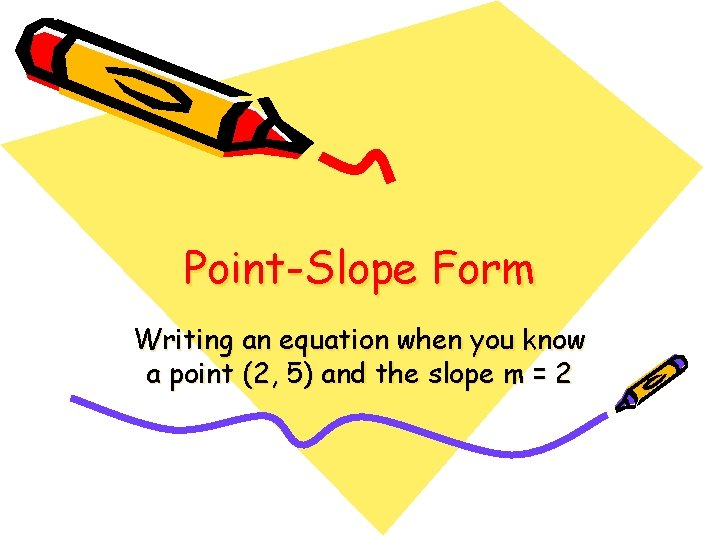Point-Slope Form Writing an equation when you know a point (2, 5) and the slope m = 2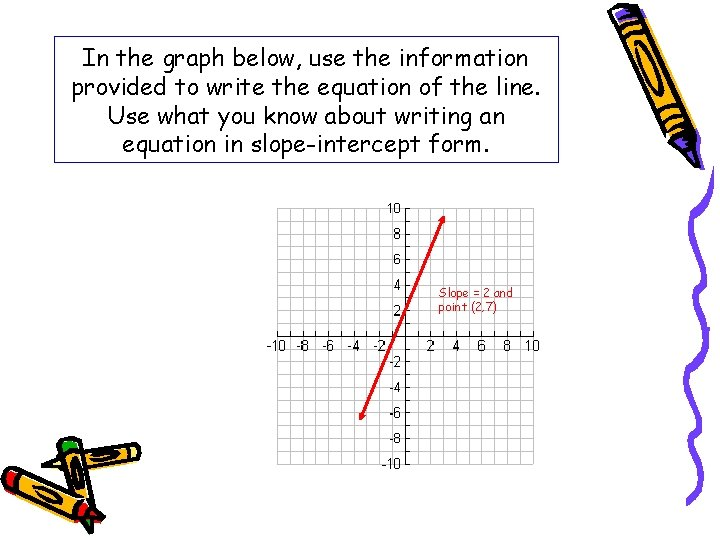In the graph below, use the information provided to write the equation of the line. Use what you know about writing an equation in slope-intercept form. Slope = 2 and point (2, 7)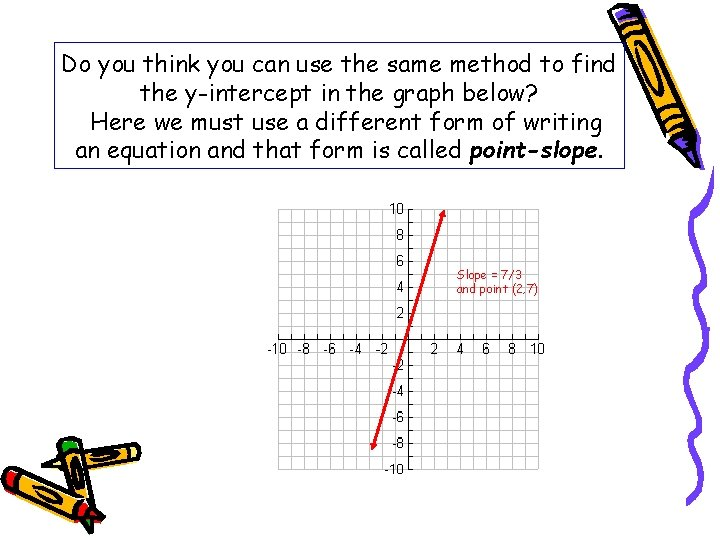Do you think you can use the same method to find the y-intercept in the graph below? Here we must use a different form of writing an equation and that form is called point-slope. Slope = 7/3 and point (2, 7)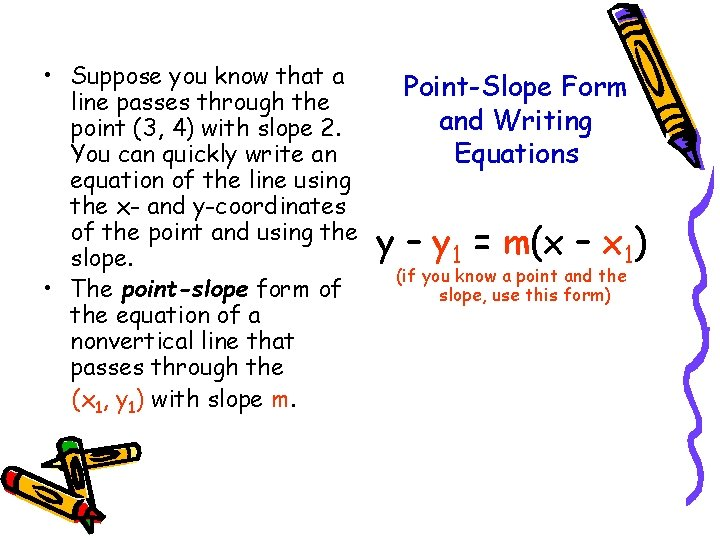• Suppose you know that a line passes through the point (3, 4) with slope 2. You can quickly write an equation of the line using the x- and y-coordinates of the point and using the slope. • The point-slope form of the equation of a nonvertical line that passes through the (x 1, y 1) with slope m. Point-Slope Form and Writing Equations y – y 1 = m(x – x 1) (if you know a point and the slope, use this form)Let’s try a couple. Using point-slope form, write the equation of a line that passes through (4, 1) with slope -2. y – y 1 = m(x – x 1) y – 1 = -2(x – 4) Substitute 4 for x , 1 for y and -2 for m. 1 Write in slope-intercept form. y – 1 = -2 x + 8 y = -2 x + 9 1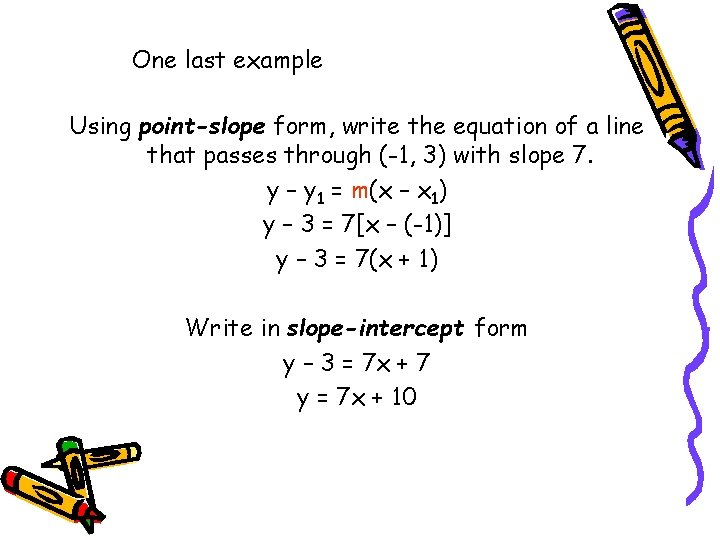One last example Using point-slope form, write the equation of a line that passes through (-1, 3) with slope 7. y – y 1 = m(x – x 1) y – 3 = 7[x – (-1)] y – 3 = 7(x + 1) Write in slope-intercept form y – 3 = 7 x + 7 y = 7 x + 10If you know two points on a line, first use them to find the slope. Then you can write an equation using either point. • Step one – Find the slope of a line with points (-4, 3), (-2, 1)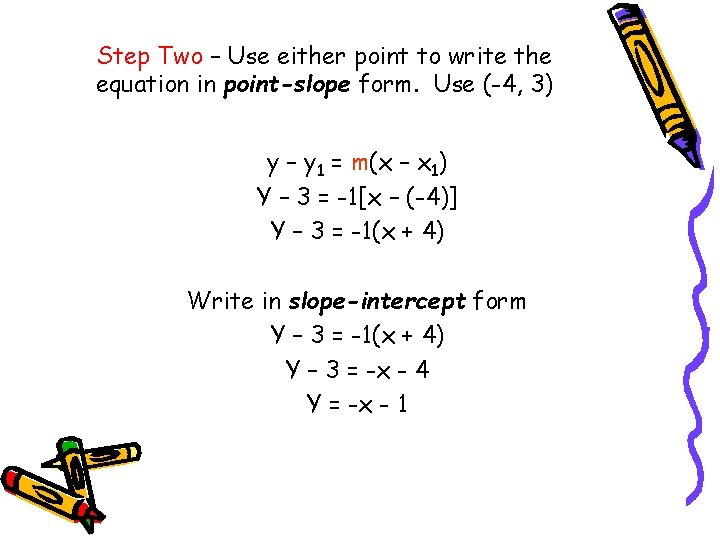Step Two – Use either point to write the equation in point-slope form. Use (-4, 3) y – y 1 = m(x – x 1) Y – 3 = -1[x – (-4)] Y – 3 = -1(x + 4) Write in slope-intercept form Y – 3 = -1(x + 4) Y – 3 = -x - 4 Y = -x - 1Writing Equations of Parallel and Perpendicular Lines Geometry ConnectionIn coordinate geometry you studied how to determine if lines where parallel or perpendicular. • Nonvertical lines are parallel if they have the same slope and different y-intercept. Any 2 vertical lines are parallel. (y = 3 x + 1 and y = 3 x -3) • Two lines are perpendicular if the product of their slopes is -1. A vertical line and a horizontal line are also perpendicular. (y = -¼x 1 and y = 4 x + 2)In the graph on the left, the two lines are parallel. Parallel lines have the same slope and never intersect. In the graph at the right, the two lines are perpendicular. Perpendicular lines are lines that intersect to form right angles.Writing Equations of Parallel Lines Write the equation for the line that contains (5, 1) and is parallel to y = ¼x – 4. Identify the slope of the given line. y = ¼x – 4 (slope is ¼) Using point-slope form, write the equation. y – y 1 = m(x – x 1) point-slope form of an equation y – 1 = ¼(x – 5) substitute (5, 1) for (x , y ) and ¼ for m Y – 1 = ¼x – 5/4 using the Distributive Property, remove the parentheses Y = ¼x – ¼ simplify and rewrite in slope-intercept The equation is y = ¼x – 1/4. 1 1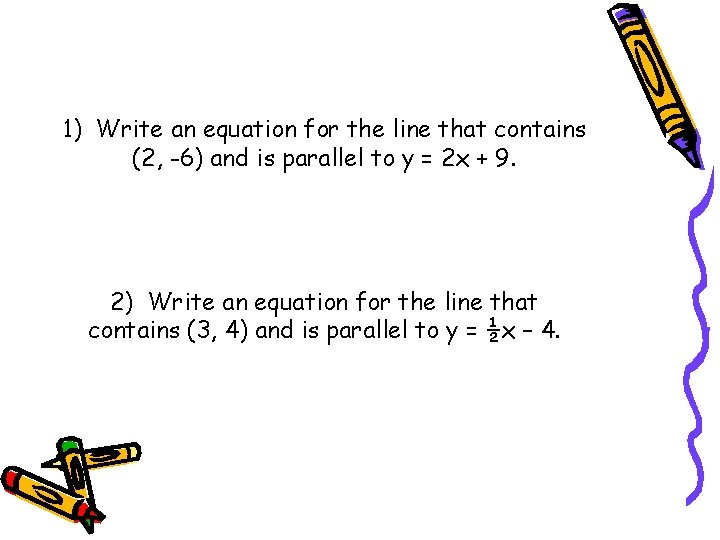1) Write an equation for the line that contains (2, -6) and is parallel to y = 2 x + 9. 2) Write an equation for the line that contains (3, 4) and is parallel to y = ½x – 4.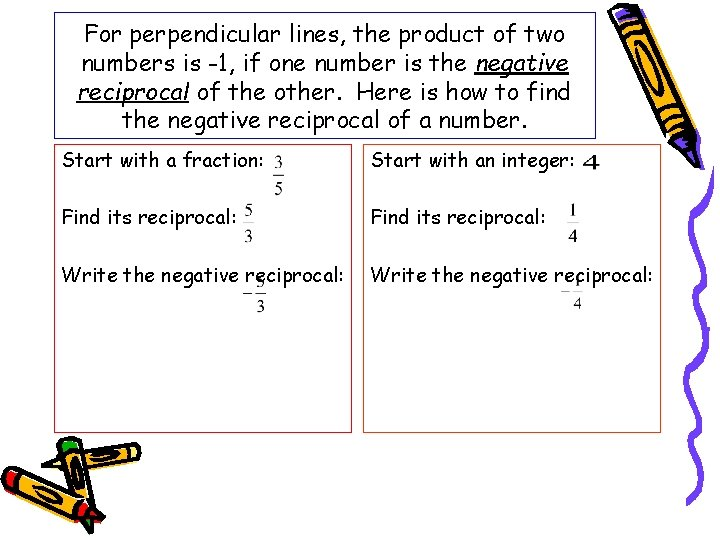For perpendicular lines, the product of two numbers is -1, if one number is the negative reciprocal of the other. Here is how to find the negative reciprocal of a number. Start with a fraction: Start with an integer: Find its reciprocal: Write the negative reciprocal: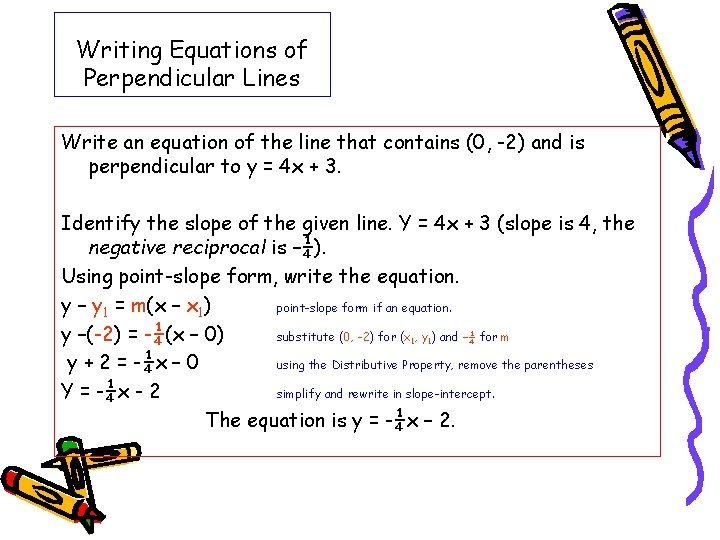Writing Equations of Perpendicular Lines Write an equation of the line that contains (0, -2) and is perpendicular to y = 4 x + 3. Identify the slope of the given line. Y = 4 x + 3 (slope is 4, the negative reciprocal is –¼). Using point-slope form, write the equation. y – y 1 = m(x – x 1) point-slope form if an equation. y –(-2) = -¼(x – 0) substitute (0, -2) for (x , y ) and –¼ for m y + 2 = -¼x – 0 using the Distributive Property, remove the parentheses Y = -¼x - 2 simplify and rewrite in slope-intercept. The equation is y = -¼x – 2. 1 1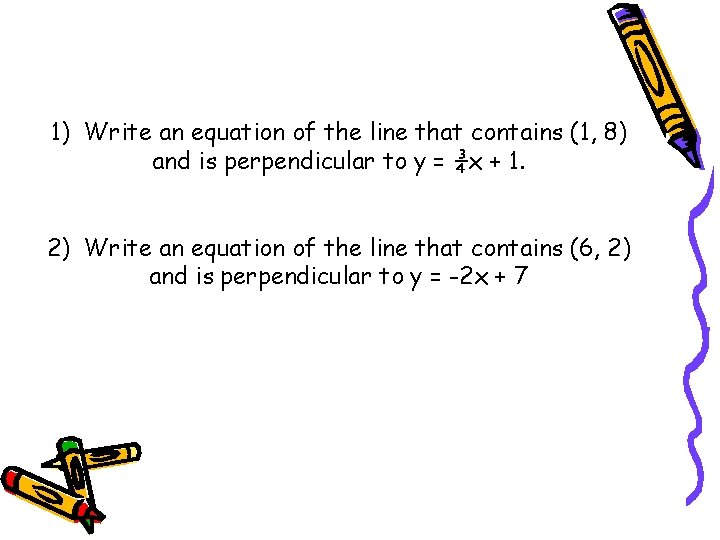1) Write an equation of the line that contains (1, 8) and is perpendicular to y = ¾x + 1. 2) Write an equation of the line that contains (6, 2) and is perpendicular to y = -2 x + 7Graphing Absolute Value Equations Distance from zero?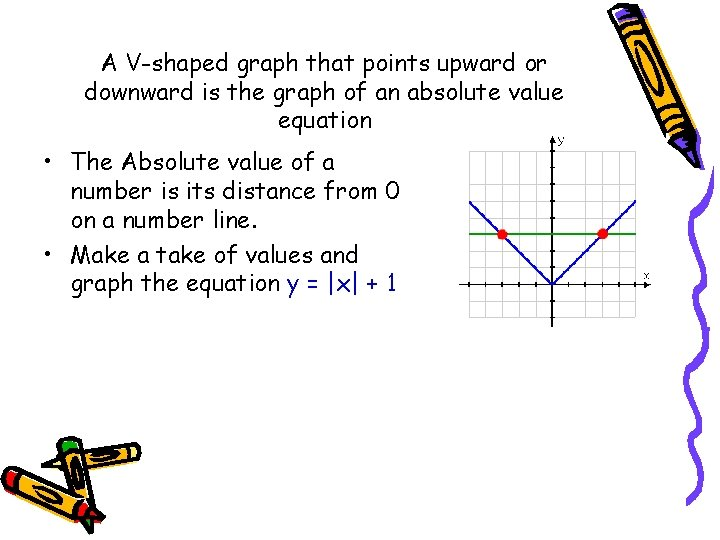A V-shaped graph that points upward or downward is the graph of an absolute value equation • The Absolute value of a number is its distance from 0 on a number line. • Make a take of values and graph the equation y = |x| + 1Below are the graphs of y = |x| + 1 and y = |x| + 2. Describe how the graphs are the same and how they are different. y = |x| + 1 y = |x| + 2 The graphs are the same shape. The y-intercept of the first graph is 1. the y-intercept of the second graph is 2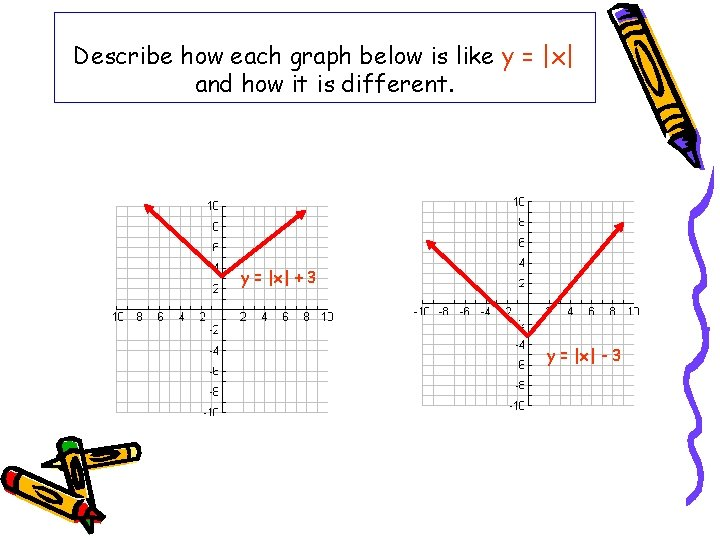Describe how each graph below is like y = |x| and how it is different. y = |x| + 3 y = |x| - 3Graph each equation (function) 1) y = |x| + 2 2) y = |x| – 4 3) y = |x| + 1 4) y = |x| - 5 Use x-values of -2, -1, 0, 1, 2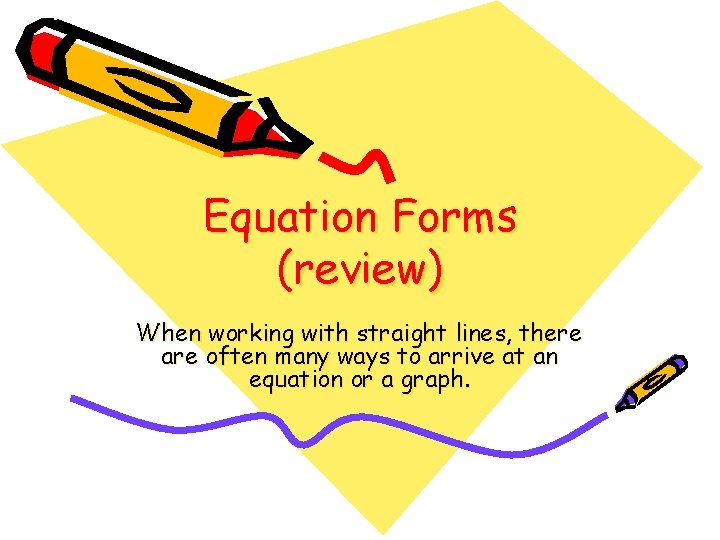Equation Forms (review) When working with straight lines, there are often many ways to arrive at an equation or a graph.Slope Intercept Form If you know the slope and where the line crosses the y-axis, use this form. y = mx + b m = slope b = y-intercept (where the line crosses the y-axis)Point Slope Form If you know a point and the slope, use this form. y – y 1 = m(x – x 1) m = slope (x 1, y 1) = a point on the lineHorizontal Lines y=3 (or any number) Lines that are horizontal have a slope of zero. They have “run” but no “rise”. The rise/run formula for slope always equals zero since rise = o. y = mx + b y = 0 x + 3 y=3 This equation also describes what is happening to the y-coordinates on the line. In this case, they are always 3.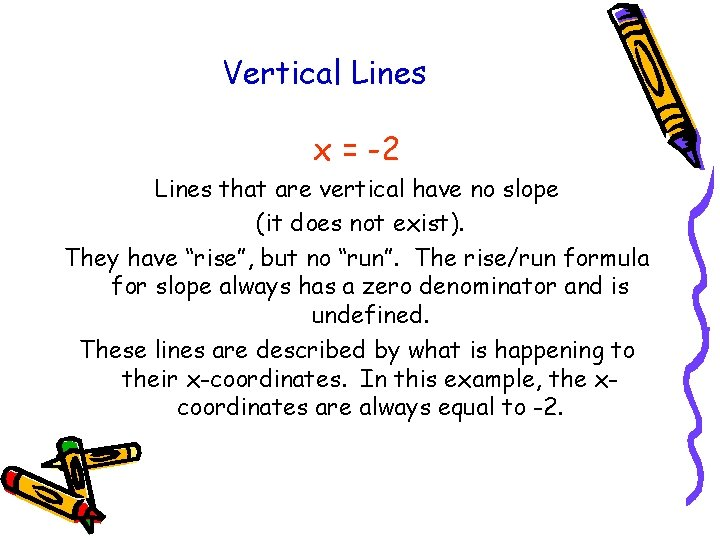Vertical Lines x = -2 Lines that are vertical have no slope (it does not exist). They have “rise”, but no “run”. The rise/run formula for slope always has a zero denominator and is undefined. These lines are described by what is happening to their x-coordinates. In this example, the xcoordinates are always equal to -2.There are several ways to graph a straight line given its equation. Let’s quickly refresh our memories on equations of straight lines: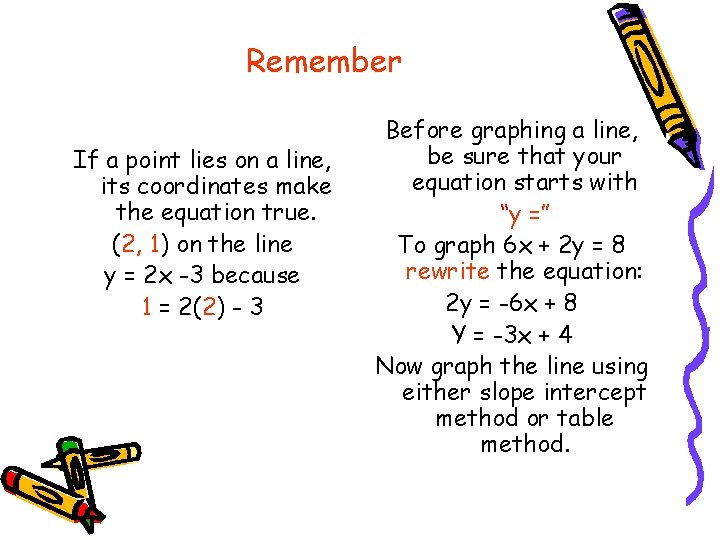Remember If a point lies on a line, its coordinates make the equation true. (2, 1) on the line y = 2 x -3 because 1 = 2(2) - 3 Before graphing a line, be sure that your equation starts with “y =” To graph 6 x + 2 y = 8 rewrite the equation: 2 y = -6 x + 8 Y = -3 x + 4 Now graph the line using either slope intercept method or table method.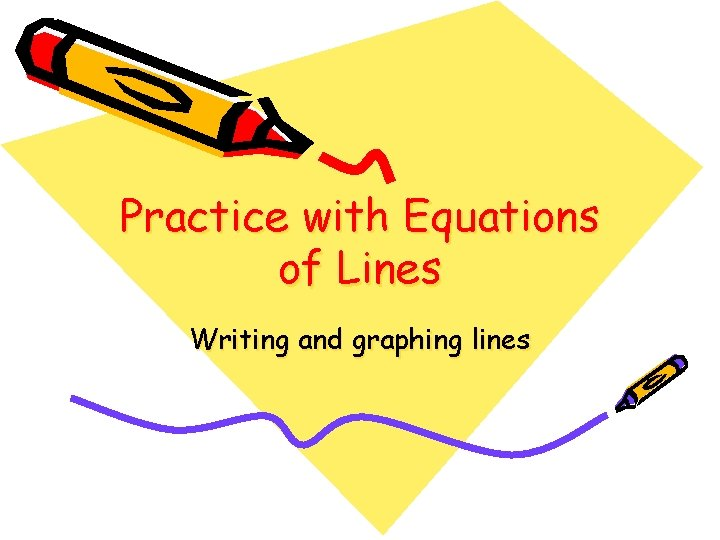Practice with Equations of Lines Writing and graphing lines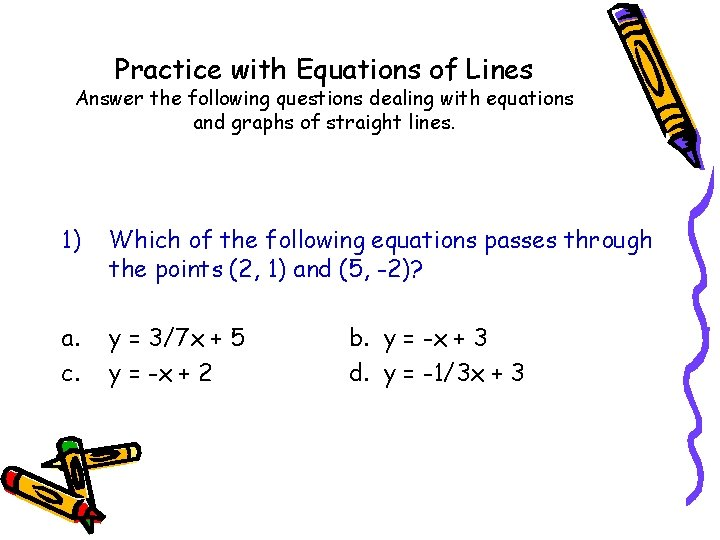Practice with Equations of Lines Answer the following questions dealing with equations and graphs of straight lines. 1) Which of the following equations passes through the points (2, 1) and (5, -2)? a. c. y = 3/7 x + 5 y = -x + 2 b. y = -x + 3 d. y = -1/3 x + 32) Does the graph of the straight line with slope of 2 and y-intercept of 3 pass through the point (5, 13)? Yes No3) The slope of this line is 3/2? True False4) What is the slope of the line 3 x + 2 y = 12? a) b) c) d) 3 3/2 -3/2 2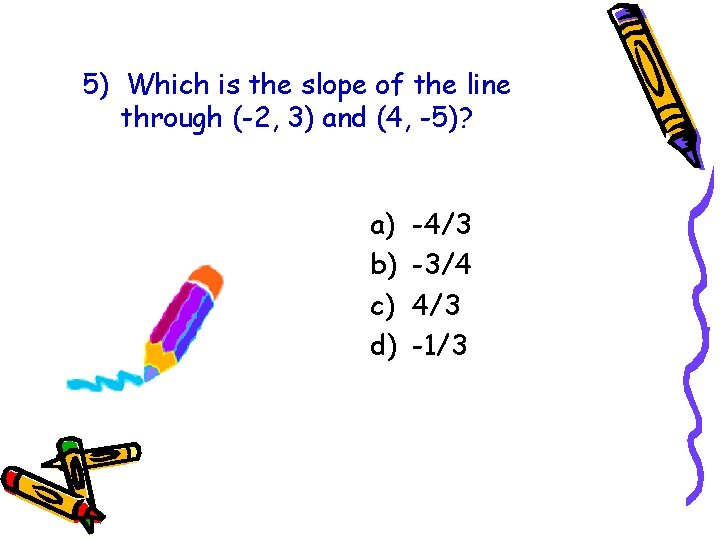5) Which is the slope of the line through (-2, 3) and (4, -5)? a) b) c) d) -4/3 -3/4 4/3 -1/36) What is the slope of the line shown in the chart below? a) b) c) d) 1 3/2 3 3/57) Does the line 2 y + x = 7 pass through the point (1, 3)? True False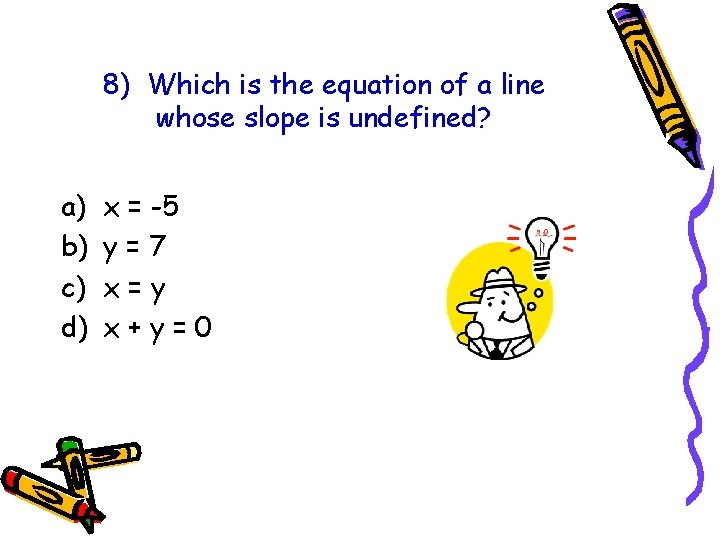8) Which is the equation of a line whose slope is undefined? a) b) c) d) x = -5 y=7 x=y x+y=09) Which is the equation of a line that passes through (2, 5) and has slope -3? a) b) c) d) y = -3 x – 3 y = -3 x + 17 y = -3 x + 11 y = -3 x + 5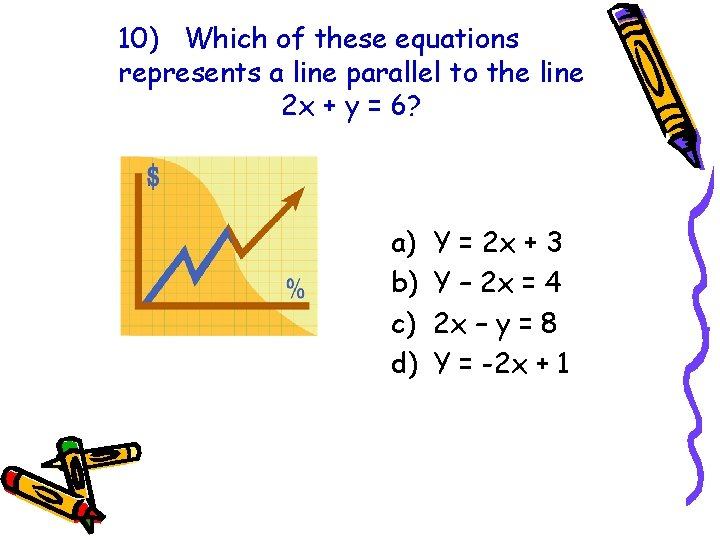10) Which of these equations represents a line parallel to the line 2 x + y = 6? a) b) c) d) Y = 2 x + 3 Y – 2 x = 4 2 x – y = 8 Y = -2 x + 1